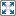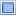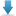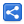# Groovy Operators - Coercion Operator

[Last Updated: Jan 23, 2019]

The coercion operator (as) converts an object from one type to another type without them being compatible for assignment.

This is different than type casting. Casting a type which is not assignable to the target type, will produce a ClassCastException at runtime. The coercion operator converts the object to the target type via predefined conversion rules even if it is not castable to the target type . Let's see examples to understand that:

#### src/Example1AsOpt.groovy

```String s = "1.1"
BigDecimal bd = s as BigDecimal;
println bd
println bd.class.name```

#### Output

`1.1java.math.BigDecimal`

In above example String is converted to `BigDecimal`. This conversion is performed in `StringGroovyMethods#asType(....)`.
To have a better idea, following is a quick snippet of `StringGroovyMethods`:

```package org.codehaus.groovy.runtime;
.....
public class StringGroovyMethods extends DefaultGroovyMethodsSupport {
....
public static <T> T asType(String self, Class<T> c) {
if (c == List.class) {
} else if (c == BigDecimal.class) {
} else if (c == BigInteger.class) {
} else if (c != Long.class && c != Long.TYPE) {
.......
}
}
........
}```

The super class `DefaultGroovyMethodsSupport` defines more asType() methods for other type conversions.

## Defining asType()

We can also define `asType(Class target)` method in our own class for as operator to work.

#### src/Example2AsOpt.groovy

```import java.math.MathContext

class Fraction {
int numerator;
int denominator;

Fraction(int numerator, int denominator) {
this.numerator = numerator
this.denominator = denominator
}

def asType(Class target) {
switch (target) {
case BigDecimal.class:
return new BigDecimal(numerator).divide(
new BigDecimal(denominator), MathContext.DECIMAL32)
case String.class:
return numerator + "/" + denominator
case Double.class:
return Double.valueOf((double) numerator / (double) denominator)
default:throw new RuntimeException("not supported: "+target);
}
}
}

def f = new Fraction(3, 11);

def bd = f as BigDecimal
println bd

def d = f as Double
println d

def s = f as String
println s
```

#### Output

`0.27272730.27272727272727273/11`

The coercion operator can also be used at other places for example during declaring types.

#### src/Example3AsOpt.groovy

```def list = [1,3,5]
println list.class.name
println list.class.isArray()

def arr = [1,3,5] as int[]
println arr.class.name
println arr.class.isArray()
```

#### Output

`java.util.ArrayListfalse[Itrue`

## Example Project

Dependencies and Technologies Used:

• Groovy 2.5.5
• JDK 9.0.1
 Groovy - Coercion OperatorSelect AllDownload• groovy-coercion-operator
• src
• Example1AsOpt.groovy

 Share